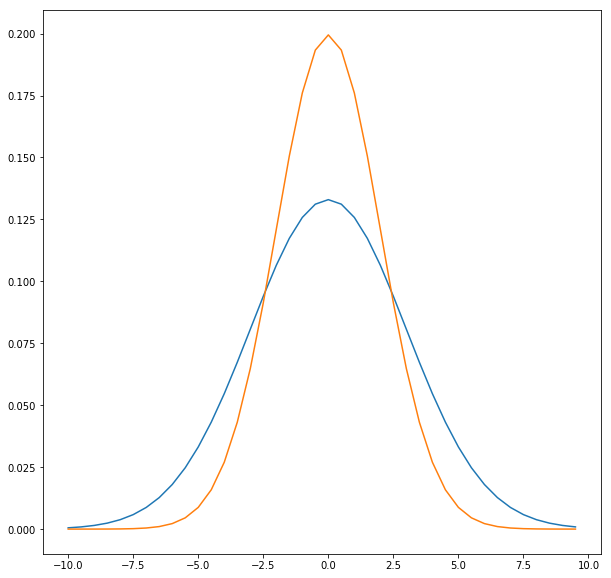# Gauss-Markov Theorem

In one of the previous posts we looked at the assumptions underlying the classic linear regression model. In this post we’ll take a look at the Gauss-Markov theorem which uses these assumptions to state that the OLS estimators are the best linear unbiased estimators, in the class of linear unbiased estimators.

## Gauss-Markov Theorem

To recap briefly, some of the assumptions we made were that:

1. On average, the stochastic error term is zero i.e. $E(u_i | X_i) = 0$.
2. The data is homoscedastic i.e. the variance / standard deviation of the stochastic error term is a finite value regardless of $X_i$.
3. The model is linear in parameters i.e. $\beta_1$ and $\beta_2$ have power 1.
4. There is no autocorrelation between the disturbance terms.

The Gauss-Markov theorem states the following:

Given the assumptions underlying the CLRM, the OLS estimators, in the class of linear unbiased estimators, have minimum variance. That is, they are BLUE (Best Linear Unbiased Estimators).

We’ll go over what it means for an estimator to be best, linear, and unbiased.## Linear

The dependent variable $Y$ is a linear function of the variables in the model. The model must be linear in parameters. Under this assumption $Y_i = \hat{\beta}_1 + \hat{\beta}_2 X_i + \hat{u}_i$ and $Y_i = \hat{\beta}_1 + \hat{\beta}_2 X_i^2 + \hat{u}_i$ are both valid models since the estimators are linear in both of them.

## Unbiased

This means that on average, the value of the estimator is the same as the parameter it is estimating i.e. $E(\hat{\beta}_1) = \beta_1$.

## Best

This means that among the class of linear estimators, the OLS estimators will have the least variance. Consider the two normal distributions shown in the graph above for two linear, unbiased estimators $\hat{\beta}_1$ (represented by orange) and $\beta_1^*$ (represented by blue). Also let’s assume that the true value of $\beta_1$ is zero. This means that both of these are unbiased since, on average, their value is the same as the true value of the parameter. However, the OLS estimator $\hat{\beta}_1$ is better since it has lesser variance compared to the other estimator.

Finito.